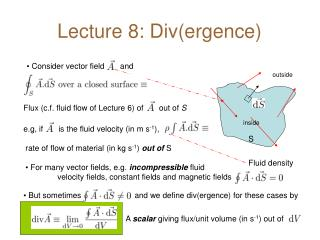DownloadDownload PresentationLecture 8: Div(ergence)

# Lecture 8: Div(ergence)

Download Presentation## Lecture 8: Div(ergence)

- - - - - - - - - - - - - - - - - - - - - - - - - - - E N D - - - - - - - - - - - - - - - - - - - - - - - - - - -
##### Presentation Transcript

1. Lecture 8: Div(ergence) • Consider vector field and outside Flux (c.f. fluid flow of Lecture 6) of out of S inside e.g, if is the fluid velocity (in m s-1), S • rate of flow of material (in kg s-1) out of S • For many vector fields, e.g. incompressible fluid velocity fields, constant fields and magnetic fields Fluid density • But sometimes and we define div(ergence) for these cases by A scalar giving flux/unit volume (in s-1) out of

2. In Cartesians • Consider tiny volume with sides dx, dy, dz so that varies linearly across it • Consider opposite areas 1 and 2 for just these two areas = dx Ax 1 dx 2 • Similar contributions from other pairs of surfaces

3. Simple Examples

4. Graphical Representation where brightness encodes value of scalar function

5. Physics Examples • Incompressible ( = constant) fluid with velocity field because for any closed surface as much fluid flows out as flows in since for any closed surface • Constant field • Compressible (  constant) fluid:  can change if material flows out/in of dV mass leaving dV per unit time A continuity equation: “density drops if stuff flows out”

6. Lines of Force • Sometimes represent vector fields by lines of force • Direction of lines is direction of • Density of lines measures • Note that div ( ) is the rate of creation of field lines, not whether they are diverging • If these field lines all close, then 2 1 1 • e.g. for magnetic field lines, there are no magnetic monopoles that could create field lines larger at 1 than 2 +Q • Electric field lines begin and end on charges: sources in dV yield positive divergence sinks in dV yield negative divergence -Q

7. Further Physics Examples (s-1) charge density (C m-3)volume (m3) current density (A m-2) surface (m2) number density (m-3) of charges drift velocity field charge of current carrier • “Charge density drops if charges flow out of dV” (continuity equation) • Maxwell’s Equations link properties of particles (charge) to properties of fields • In quantum mechanics, similar continuity equations emerge from the Schrödinger Equation in which  becomes the probability density of a particle being in a given volume and becomes a probability current